# MHD Generalized Couette Flow and Heat Transfer on Bingham Fluid Through Porous Parallel Plates

MHD Generalized Couette Flow and Heat Transfer on Bingham Fluid Through Porous Parallel Plates

Md Tusher MollahMuhammad Minarul Islam Sheela Khatun Md Mahmud Alam

Department of Mathematics, Faculty of Science, Bangabandhu Sheikh Mujibur Rahman Science & Technology University, Gopalganj 8100, Bangladesh

Mathematics Discipline, Science, Engineering and Technology School, Khulna University, Khulna 9208, Bangladesh

Corresponding Author Email:
tusher.bsmrstu@gmail.com
Page:
483-490
|
DOI:
https://doi.org/10.18280/mmep.060402
12 June 2019
|
Accepted:
18 September 2019
|
Published:
24 December 2019
| Citation

OPEN ACCESS

Abstract:

Bingham fluid through porous parallel plates with Ion-slip and Hall currents has been studied numerically. The non-linear PDEs, governing the problem under assumptions, have been transformed into dimensionless non-linear PDEs by using usual transformations. The obtained dimensionless governing equations have been solved numerically by applying the explicit finite difference method (FDM) with the help of MATLAB R2015a tool. The time sensitivity test is performed for the steady-state solution and is obtained at dimensionless time τ=4.00. It is also observed that the secondary velocity reaches the steady-state more gradually than the primary velocity and temperature profiles. The appropriate mesh space (m=40 and n=40) is obtained by the mesh sensitivity test. The impact of various interesting parameters on the primary velocity, secondary velocity and temperature profiles, also on the local Nusselt number and shear stress have been analyzed and discussed through the graph in details. Finally, a qualitative and quantitative comparison with the published results has been discussed.

Keywords:

MHD flow, Bingham fluid, generalized Couette flow, heat transfer, finite difference method (FDM)

1. Introduction

The field of magneto-hydrodynamics (MHD) has been concerned with geophysical and astrophysical problems for several numbers of years. The consideration of combined heat transfer arises due to buoyancy forces investigated by thermal diffusions. To affect a flowing stream of an electrically conducting fluid the MHD is used and which is for the resolution of thermal protection, braking, propulsion, and control. The MHD Bingham fluid flows are used in many geological and industrial materials as a common mathematical model of, mud flow in drilling engineering, handling of painting oil, cement, slurries, lava, etc. The Bingham fluid is a special class of viscoplastic fluid that begins to flow after certain stress and that exhibits a linear performance of shear stress against shear rate. The inventor of Bingham fluid is E.C. Bingham , in 1916 who described it. In recent times, the Bingham fluid with or without the magnetic field, Hall current, suction or injection, and flowing through parallel plates have been examined by several characters.

In this regard, an exact description of friction loss for Bingham plastics through laminar pipe flow has been first published by Buckingham . The physical and chemical properties of the Bingham fluid have been described by Bingham . The general boundary layer equations of Bingham fluid for continuous surfaces have been analyzed by Sakiadis . The flow past a continuously moving surface such as hot rolling, metal and plastic extrusion, glass fiber, continuous casting including paper production, the steady heat transfer flow beyond a continuously moving plate with variable temperature have been analyzed by Soundalgekar and Murty . Darby and Melson  developed an experimental formulation to prophesy the friction factor for a flow of Bingham plastics. Attia and Sayed-Ahmed  studied the influences of Hall current on unsteady MHD laminar Couette flow and heat transfer on Bingham fluid with Hall Current, suction and injection. The slow spreading of a sheet of Bingham fluid on an inclined plane has been established by Liu and Mei . Naik et al.  studied the effect of Hall current on the MHD free connective laminar Couette flow of Bingham fluid with thermal radiation. The unsteady viscous incompressible MHD Bingham fluid flowing through parallel plates with hall current and suction has been studied by Parvin et al. . Rees and Bassom  discussed the unsteady flows of a Bingham fluid in a porous medium. The influence of Hall and Ion-slip currents MHD free convection and mass transfer flow through a vertical oscillatory porous plate in a rotating porous medium with heat source has been investigated by Hossain et al. . Islam et al.  mentioned the comparison criteria of the obtained result with the published result. Mollah et al. [14, 15] studied the Hall and Ion-slip effects on unsteady MHD Bingham fluid flow with suction. They also discussed the Bingham fluid flow in different cases, like, considering the laminar flow between two Riga (a combination of stable magnets and alternating electrodes) plates , considering oscillation on the upper plate of the porous channel . Islam et al.  studied the Bingham fluid through parallel plate, they didn’t consider the magnetohydrodynamic (MHD) phenomena while in previous research the MHD phenomena are considered [14-17].

Now the question arises: What are the flow characteristics while the Bingham fluid is flowing through a porous parallel plate in the presence of Hall and Ion-slip current? In the present study, in order to answer this question, the numerical study has been performed to discuss the MHD generalized Couette flow and heat transfer on Bingham fluid through porous parallel plates with Ion-slip and Hall currents. The governing equations are concerned with the generalized Ohm’s law including Hall and Ion-slip currents, porous medium and Rheology of the Bingham fluid. The explicit finite difference technique has been used to find out the solution of dimensionless governing PDEs. The obtained outcomes have been shown in the graph.

2. Mathematical Model

Figure 1 describes the physical configuration of the study, where the viscous incompressible flow of Bingham fluid is considered to be flowing among a couple of infinite parallel porous plates which are positioned at $y=\pm h\,$ planes and lengthen from $x=0$ to $\infty . U_{0}$ be the uniform velocity at which the upper plate is moving and the rest plate is motionless. ${{T}_{2}}$ be the temperature of upper plate and ${{T}_{1}}$ temperature is for the lower plate, where ${{T}_{2}}>{{T}_{1}}$. A constant pressure gradient $\frac{d p}{d x}$ is emploied on the fluid along the X-direction and the body force is neglected. A uniform magnetic field ${{B}_{0}}$ is applied along the Y-direction which is undisturbed as the induced magnetic field is ignored by considering a very small magnetic Reynolds number. Due to the attention of Hall Effect, a Z-element for the velocity is supposed to begin. Thus, the fluid velocity vector is given as $\mathbf{\tilde{q}}=\tilde{u}\mathbf{i}+\tilde{v}\mathbf{j}+\tilde{w}\mathbf{k}$.

## 1.jpg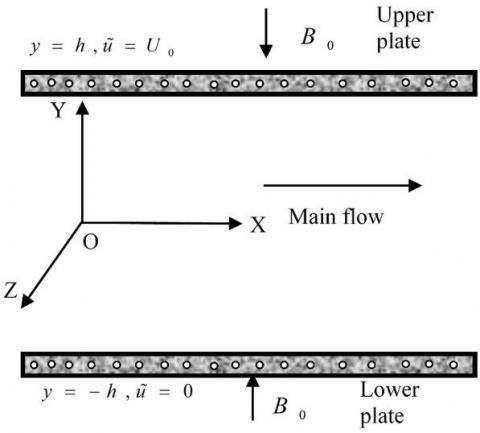Figure 1. The physical configuration of the problem

Within the basis of the above assumptions, the equations, for this unsteady 2D problem, are governed by the system of coupled non-linear PDEs, are given in vector form as follows:

Continuity Equation:

$\nabla .\mathbf{\tilde{q}}=0$                                 (1)

Momentum Equation:

$\frac{\partial \mathbf{\tilde{q}}}{\partial t}+(\mathbf{\tilde{q}}.\nabla )\mathbf{\tilde{q}}=-\frac{1}{\rho }\nabla p+\frac{1}{\rho }\nabla .\left( \tilde{\mu }\nabla \mathbf{\tilde{q}} \right)+\frac{1}{\rho }\left( \mathbf{J}\wedge \mathbf{B} \right)-\frac{\upsilon }{k'}\mathbf{\tilde{q}}$            (2)

Energy Equation:

$\frac{\partial \tilde{T}}{\partial t}+(\mathbf{\tilde{q}}.\nabla )\tilde{T}=\frac{\kappa }{\rho {{c}_{p}}}{{\nabla }^{2}}\tilde{T}+\frac{1}{\rho {{c}_{p}}}\Phi +\frac{1}{\rho {{c}_{p}}\sigma '}{{J}^{2}}$                    (3)

where, $\tilde{\mu }=K+\frac{{{\tau }_{0}}}{\sqrt{{{\left( \frac{\partial \tilde{u}}{\partial y} \right)}^{2}}+{{\left( \frac{\partial \tilde{w}}{\partial y} \right)}^{2}}}}$,

$\Phi =\tilde{\mu }\left[ 2\left\{ {{\left( \frac{\partial \tilde{u}}{\partial x} \right)}^{2}}+{{\left( \frac{\partial \tilde{v}}{\partial y} \right)}^{2}} \right\}+{{\left( \frac{\partial \tilde{u}}{\partial y}+\frac{\partial \tilde{v}}{\partial x} \right)}^{2}}+{{\left( \frac{\partial \tilde{w}}{\partial y} \right)}^{2}}+{{\left( \frac{\partial \tilde{w}}{\partial x} \right)}^{2}} \right]$, $\mathbf{J}\wedge \mathbf{B}=-\frac{{{\sigma }_{\text{e}}}B_{_{0}}^{2}}{\alpha _{\text{e}}^{2}+\beta _{\text{e}}^{2}}\left[ {{\alpha }_{e}}\tilde{u}+{{\beta }_{e}}\tilde{w} \right]\mathbf{i}+\frac{{{\sigma }_{\text{e}}}B_{_{0}}^{2}}{\alpha _{\text{e}}^{2}+\beta _{\text{e}}^{2}}\left[ {{\beta }_{e}}\tilde{u}-{{\alpha }_{e}}\tilde{w} \right]\mathbf{k}$,

${{\alpha }_{e}}=1+{{\beta }_{i}}{{\beta }_{e}}$ and ${{J}^{2}}=\frac{\sigma _{\text{e}}^{\text{2}}B_{_{0}}^{2}}{{{\left( \alpha _{\text{e}}^{2}+\beta _{\text{e}}^{2} \right)}^{2}}}\left( {{{\tilde{u}}}^{2}}+{{{\tilde{w}}}^{2}} \right)$

Also the corresponding conditions can be expresssed as follows:

$t\le 0,\,\,\,\,\,\tilde{u}=0,\,\,\text{ }\tilde{w}=0,\,\,\text{ }\,\tilde{T}={{T}_{1}}$     everywhere  \begin{align} & \,\,\,\,\,\,\,\,\,\,\,\,\,\,\,\,\,\,\tilde{u}\to 0,\,\text{ }\tilde{w}=0,\,\,\,\,\,\,\,\,\text{ }\tilde{T}={{T}_{1}}\,\,\,\,\,\,\,\,\,\text{at}\,\,\text{x=0} \\ & t>0,\,\,\,\,\text{ }\tilde{u}=0,\,\,\text{ }\tilde{w}=0,\,\,\,\,\,\,\,\text{ }\,\tilde{T}={{T}_{1}}\,\,\,\,\,\,\,\,\,\text{at}\,\,y=-h \\ & \,\,\,\,\,\,\,\,\,\,\,\,\,\,\,\,\,\tilde{u}={{U}_{0}},\,\text{ }\tilde{w}=0,\,\,\,\,\text{ }\tilde{T}={{T}_{2}}\,\,\,\,\,\,\,\,\,\,\text{at}\,\,\,y=h \\ \end{align}

It is required to transform the above governing equations into dimensionless form, as the solution of Eqns. (1 to 3) subject to the above conditions will be based on the finite difference scheme for numerical solution. The dimensionless quantities that have been used are given as follows:

\begin{align} & X=\frac{x}{h},Y=\frac{y}{h},U=\frac{{\tilde{u}}}{{{U}_{0}}},V=\frac{{\tilde{v}}}{{{U}_{0}}},W=\frac{{\tilde{w}}}{{{U}_{0}}}, \\ & P=\frac{p}{\rho U_{0}^{2}},\tau =\frac{t{{U}_{0}}}{h},\theta =\frac{\tilde{T}-{{T}_{1}}}{{{T}_{2}}-{{T}_{1}}},\bar{\mu }=\frac{{\tilde{\mu }}}{K} \\ \end{align}              (4)

Also the dimensionless quantities are given as follows:

Bingham number or dimensionless yield stress, $\tau_{D}=\frac{\tau_{0} h}{K U_{0}}$; Reynolds number, $R_{e}=\frac{\rho U_{0} h}{K}$; Prandtl number, $P_{r}=\frac{\rho c_{p} U_{0} h}{k}$ and Eckert number, $E_{C}=\frac{U_{0} K}{\rho c_{p} h\left(T_{2}-T_{1}\right)}$;

Hartmann number, $H a=\sqrt{\frac{\sigma}{K}} B_{0} h$ and

Permeability of porous medium, $k_{0}=\frac{v^{2}}{k U_{0}^{2}}$

The obtained dimensionless non-linear PDEs are given as follows:

$\frac{\partial U}{\partial X}+\frac{\partial V}{\partial Y}=0$                           (5)

\begin{align} & \frac{\partial U}{\partial \tau }+U\frac{\partial U}{\partial X}+U\frac{\partial V}{\partial Y}=-\frac{dP}{dX}-{{k}_{0}}U \\ & \text{ }+\frac{1}{{{R}_{e}}}\left[ \frac{\partial }{\partial Y}\left( \bar{\mu }\frac{\partial U}{\partial Y} \right)-\frac{H_{a}^{2}}{\alpha _{\text{e}}^{2}+\beta _{\text{e}}^{2}}\left( {{\alpha }_{e}}U+{{\beta }_{e}}W \right) \right] \\ \end{align}                        (6)

\begin{align} & \frac{\partial W}{\partial \tau }+U\frac{\partial W}{\partial X}+U\frac{\partial W}{\partial Y}=-{{k}_{0}}W \\ & \text{ +}\frac{1}{{{R}_{e}}}\left[ \frac{\partial }{\partial Y}\left( \bar{\mu }\frac{\partial W}{\partial Y} \right)-\frac{H_{a}^{2}}{\alpha _{\text{e}}^{2}+\beta _{\text{e}}^{2}}\left( {{\alpha }_{e}}W-{{\beta }_{e}}U \right) \right] \\ \end{align}                               (7)

\begin{align} & \frac{\partial \theta }{\partial \tau }+U\frac{\partial \theta }{\partial X}+\frac{\partial \theta }{\partial Y}=\frac{1}{{{P}_{r}}}\frac{{{\partial }^{2}}\theta }{\partial {{Y}^{2}}}+{{E}_{c}}\bar{\mu }\left[ {{\left( \frac{\partial U}{\partial Y} \right)}^{2}}+{{\left( \frac{\partial W}{\partial Y} \right)}^{2}} \right] \\ & \text{ }+\frac{{{E}_{c}}H_{a}^{2}}{\left( \alpha _{\text{e}}^{2}+\beta _{\text{e}}^{2} \right)}\cdot \left[ {{U}^{2}}+{{W}^{2}} \right] \\ \end{align}                                            (8)

$\bar{\mu }=1+\frac{{{\tau }_{D}}}{\sqrt{{{\left( \frac{\partial U}{\partial Y} \right)}^{2}}+{{\left( \frac{\partial W}{\partial Y} \right)}^{2}}}}$                           (9)

And the dimensionless initial and boundary conditions are given as follows:

$\tau \le 0,\,\,\,\,\,U=0,W=0,\theta =0$     everywhere                    (10)

\begin{align} & \,\,\,\,\,\,\,\,\,\,\,\,\,\,\,\,\,U\to 0,W=0,\theta =0\,\,\,\,\text{at}\,\,X\text{=0} \\ & \tau >0,\text{ }U=0,W=0,\theta =0\,\,\,\,\text{at}\,\,Y=-1 \\ & \,\,\,\,\,\,\,\,\,\,\,\,\,\,\,\,\,U=1,W=0,\theta =1\,\,\,\,\text{at}\,\,\,Y=1 \\ \end{align}                              (11)

Furthermore, the shear stress at moving plate has been studied from the velocity profile. The local shear stress in X-direction for moving plate is $\tau_{w} \equiv[\bar{\mu} \sqrt{\left(\frac{\partial U}{\partial Y}\right)^{2}+\left(\frac{\partial W}{\partial Y}\right)^{2}}]_{Y=1}$.

Also the Nusselt number at moving plate has been studied from the temperature profile. The local Nusselt number in X-direction for moving plate is $N_{u} \equiv \frac{\left(\frac{\partial T}{\partial Y}\right)_{Y=1}}{{-\left(T_{m}-1\right)}}$; where, $T_{m}=\frac{\int_{-1}^{1} U \theta d Y}{\int_{-1}^{1} U d Y}$.

3. Numerical Technique

The finite difference method (FDM) is chosen to solve the dimensionless system (5-9) subject to the boundary conditions (10 and 11). Many scholars use the same FDM numerical technique [19, 20], as of them the division is made inside the area of boundary layer by the lines perpendicular to axes to make the wel-known mesh space as shown in Figure 2.

Consider the maximum length of the plate ${{X}_{\max }}\left( =40 \right)$ i.e. for the numerical calculation, dimensionless length X ranges from 0 to 40. Since the boundary condition shows that the plates are placed at $Y=-1$ and $Y=1$ i.e. the distance between two plates ${{Y}_{\max }}=2$, thus for the numerical calculation, dimensionless lenght Y ranges from 0 to 2. Consider suitable m and n known as number of grid spacing in the X and Y directions respectively. The suitable values are choosen (m,n)=(40,40), see the section 5.1. It is assumed that $\Delta X$ and $\Delta Y$ are constant mesh sizes along X and Y directions respectively and taken as follows, $\Delta X={{{X}_{\max }}}/{m=}\;1.0\,\left( 0\le X\le 40 \right)$ and $\Delta Y={{{Y}_{\max }}}/{n=}\;0.05\,\left( 0\le Y\le 2 \right)$ with the smaller time-step, $\Delta \tau =0.0001$ (chosen arbitrarily small).

## 2.jpgFigure 2. Finite difference grid space

Within the above discussed values the FDM calculation is performed by the MATLAB 2015a tool to achive the steady-state solution which is described in the later section.

4. Results and Discussion

To evaluate the physical configuration of the developed problem, the numerical values have been computed for the dimensionless primary velocity (U), secondary velocity (W) and temperature $\left( \theta \right)$ distributions inside the boundary layer. First of all, the appropriate grid space is computed (see section 4.1) and the grid space, (m,n)=(40,40) can be chosen as the appropriate mesh size.  Then the steady-state solution is calculated for the velocity and temperature profiles (see section 4.2), and is obtained for the dimensionless time $\tau =$4.00. After that; the impact of Hall parameter $\left(\beta_{e}\right)$ Ion-slip parameter $\left(\beta_{i}\right)$ and Reynolds number $\left( {{R}_{e}} \right)$ on velocity including temperature distributions also on the local shear stress $\left(\tau_{w}\right)$ and local Nusselt number $\left(N_{u}\right)$ at the moving plate are discussed in section 4.3. For brevity, the effect of other parameters such as Hartmann number $\left(H_{a}\right)$, Prandtl number $\left(P_{r}\right)$, Eckert number $\left(E_{c}\right)$, Permeability of porous medium $\left(k_{0}\right)$ and Bingham number $\left(\tau_{D}\right)$ are not shown. Finally, a qualitative and quantitative comparison of the current study with the published results of Mollah et al.  has been discussed in section 4.4.

4.1 Calculating appropriate grid space

The mesh sensitivity test is applied here. To obtain the appropriate grid space i.e. to obtain the appropriate values of m and n the calculations have been continued for  three different grid spaces such as (m,n)=(40,40); (m,n)=(60,60) and (m,n)=(80,80) (see Figure 3), where ${{\beta }_{e}}=\text{3}.00,$ ${{\beta }_{i}}=\,\text{3}.00,$ ${{H}_{a}}=\,\text{3}.00,$ ${{R}_{e}}=\,\text{3}.00,$ ${{E}_{c}}=0.01,$ ${{P}_{r}}=\,0.\text{30,}$ ${{k}_{0}}=\,0.50$ and ${{\tau }_{D}}=\,0.10$. The obtained curves are smooth and shows negligible changes between them. Thus, (m,n)=(40,40) can be chosen as the appropriate mesh size. According to this situation, the further calculation have been carried out for the mesh size (m,n)=(40,40).

## 3.jpg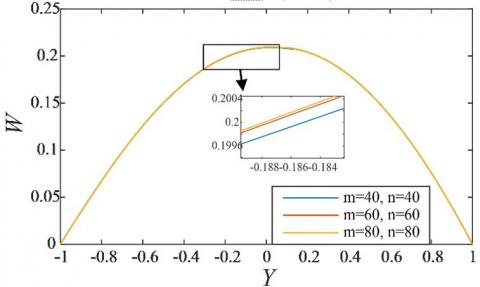Figure 3. Justifying grid space

## 4.jpg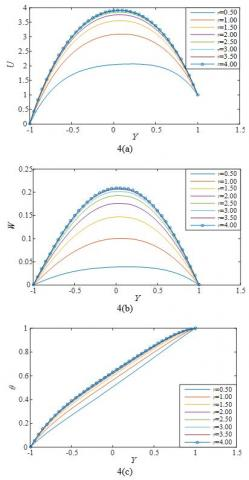Figure 4. Illustration of time variation for (a) Primary velocity, (b) Secondary velocity and (c) Temperature distributions, where ${{\beta }_{e}}=\text{3}.00,$ ${{\beta }_{i}}=\,\text{3}.00,$ ${{H}_{a}}=\,\text{3}.00,$ ${{R}_{e}}=\,\text{3}.00,$ ${{E}_{c}}=0.01,$ ${{P}_{r}}=\,0.\text{30,}$ ${{k}_{0}}=\,0.50$ and ${{\tau }_{D}}=\,0.10$

The time sensitivity test is applied here. To find out the steady state values of this research, the calculations have been continued for eight different time step sizes such as $\tau =$ 0.50, 1.00, 1.50, 2.00, 2.50, 3.00, 3.50 and 4.00. It is observed that, the result of computations for different profiles shows negligible changes after $\tau =$3.50. Thus the solutions for all variables at dimensionless time $\tau =$4.00 are taken as the steady-state solutions. The time illustrations for primary, secondary velocity and temperature distributions are shown in Figure 4.

Figure 4 shows that the primary velocity, secondary velocity and temperature profiles reach their steady state monotonically. The secondary velocity reaches the steady state more gradually than the primary velocity and temperature profiles.

4.3 Effect of parameters

To clarify the conception of physical configuration of this research, the effects of some important parameters on velocity and temperature distributions also on the local shear stress and local Nusselt number at moving plate are discussed graphically. The effects are discussed for  Hall parameter $\left( {{\beta }_{e}} \right)$, Ion-slip parameter $\left( {{\beta }_{i}} \right)$, and Reynolds number$\left( {{R}_{e}} \right)$ in the presence of Hartmann number ($H_{a}=3.00$), Prandtl number $\left(P_{r}=0.30\right)$, Eckert number $\left(H_{a}=3.00\right)$, Permeability of porous medium $\left(k_{0}=0.50\right)$ and Bingham number $\left(\tau_{D}=0.10\right)$, see Figures 5 to 10. Where the constant values of different parameters are chosen by trial and error mechanism that gives the best fit.

The effect of Hall Parameter $\left( {{\beta }_{e}} \right)$ on primary velocity, secondary velocity, temperature distributions also on the local shear stress including local Nusselt number at moving plate have shown in Figures 5 and 6. From Figure 5(a,b,c),  it is observed that the primary velocity and temperature profiles increases with the increase of ${{\beta }_{e}}$ while the secondary velocity profile decreases with the increment of ${{\beta }_{e}}$. Furthermore, the Figure 6(a,b), shows that  the local Nusselt number decreases with the increment of ${{\beta }_{e}}$ while the local shear stress increases with the increase of ${{\beta }_{e}}$ at moving plate.

Again, the effect of Ion-slip parameter $\left( {{\beta }_{i}} \right)$ on primary velocity, secondary velocity also temperature distributions as well as local shear stress including local Nusselt number at moving plate have shown in Figures 7 and 8. From Figure 7(a,b,c), it is observed the primary velocity and temperature profiles increases with the raise of ${{\beta }_{i}}$ while the secondary velocity profile decreases with the increment of ${{\beta }_{i}}$. Also, the Figure 8(a,b), shows that  the Nusselt number decreases with the increment of ${{\beta }_{i}}$ elsewhere the local shear stress increases with the raise of ${{\beta }_{i}}$ at moving plate.

Furthermore, the effect of Reynolds number ${{R}_{e}}$ on primary velocity, secondary velocity hereafter temperature distributions as well as local shear stress and local Nusselt number at moving plate have displayed in Figures 9 and 10. From Figure 9(a,b,c), it is found that the primary velocity, secondary velocity also temperature profiles increases with the raise of ${{R}_{e}}$. It is observed from Figure 10(a,b), that the local Nusselt number decreases with the raise of ${{R}_{e}}$ while the local shear stress increases with the forward movement of ${{R}_{e}}$ at moving plate.

## 5.jpg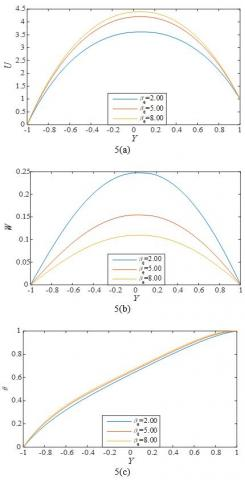Figure 5. Effects of Hall Parameter $\left( {{\beta }_{e}} \right)$ on (a) Primary velocity; (b) Secondary velocity and (c) Temperature distributions; where, ${{\beta }_{i}}=\,\text{3}.00,$ ${{H}_{a}}=\,\text{3}.00,$ ${{R}_{e}}=\,\text{3}.00,$ ${{E}_{c}}=0.01,$ ${{P}_{r}}=\,0.\text{30,}$ ${{k}_{0}}=\,0.50$ and ${{\tau }_{D}}=\,0.10$ at time $\tau =4.00$ (Steady State)

Figure 5 shows that the primary velocity and temperature profiles increases with the raise of  ${{\beta }_{e}}$ while the secondary velocity profile decreases with the increment of ${{\beta }_{e}}$.

Figure 6 shows that the local Nusselt number decreases with the increment of ${{\beta }_{e}}$ at moving plate while the local shear stress increases with the raise of ${{\beta }_{e}}$ at moving plate.

## 6.jpg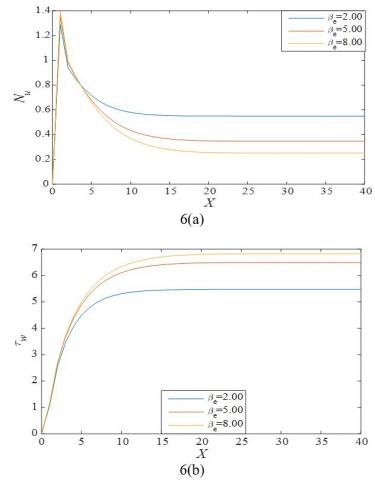Figure 6. Effects of Hall Parameter $\left( {{\beta }_{e}} \right)$ on (a) local Nusselt number and (b) local shear stress at moving plate; where,${{\beta }_{i}}=\,\text{3}.00,$ ${{H}_{a}}=\,\text{3}.00,$ ${{R}_{e}}=\,\text{3}.00,$ ${{E}_{c}}=0.01,$ ${{P}_{r}}=\,0.\text{30,}$ ${{k}_{0}}=\,0.50$ and ${{\tau }_{D}}=\,0.10$ at time$\tau =4.00$(Steady State)

## 7.jpgFigure 7. Effects of Ion-slip Parameter $\left( {{\beta }_{i}} \right)$ on (a) Primary velocity; (b) Secondary velocity and (c) Temperature distributions; where, ${{\beta }_{e}}=\text{3}.00,$ ${{H}_{a}}=\,\text{3}.00,$ ${{R}_{e}}=\,\text{3}.00,$ ${{E}_{c}}=0.01,$ ${{P}_{r}}=\,0.\text{30,}$ ${{k}_{0}}=\,0.50$ and ${{\tau }_{D}}=\,0.10$ at time$\tau =4.00$ (Steady State)

Figure 7 shows that the primary velocity and temperature profiles increases with the increase of $\beta_{i}$ while the secondary velocity profile decreases with the increment of $\beta_{i}$.

## 8.jpgFigure 8. Effects of Ion-slip Parameter $\left(\beta_{i}\right)$ on (a) local Nusselt number and (b) local shear stress at moving plate; where, ${{\beta }_{e}}=\text{3}.00,$ ${{H}_{a}}=\,\text{3}.00,$ ${{R}_{e}}=\,\text{3}.00,$ ${{E}_{c}}=0.01,$ ${{P}_{r}}=\,0.\text{30,}$ ${{k}_{0}}=\,0.50$ and ${{\tau }_{D}}=\,0.10$ at time$\tau =4.00$ (Steady State)

Figure 8 shows that the local Nusselt number decreases with the rise of ${{\beta }_{i}}$ at moving plate while the local shear stress increases with the raise of ${{\beta }_{i}}$ at moving plate.

## 9.jpg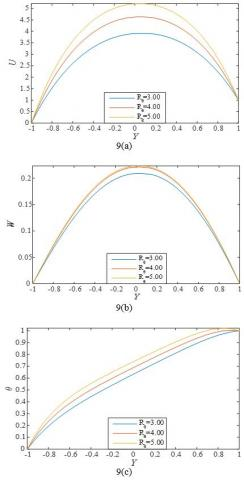Figure 9. Effects of Reynolds number $\left(R_{e}\right)$ on (a) Primary velocity; (b) Secondary velocity and (c) Temperature distributions; where, ${{\beta }_{e}}=\text{3}.00,$ ${{H}_{a}}=\,\text{3}.00,$${{\beta }_{i}}=\,\text{3}.00, {{E}_{c}}=0.01,$${{P}_{r}}=\,0.\text{30,}$ ${{k}_{0}}=\,0.50$ and ${{\tau }_{D}}=\,0.10$ at time$\tau =4.00$ (Steady State)

## 10.jpgFigure 10. Effects of Reynolds number $\left( {{R}_{e}} \right)$ on (a) local Nusselt number and (b) local shear stress at moving plate; where, ${{\beta }_{e}}=\text{3}.00,$ ${{H}_{a}}=\,\text{3}.00,$ ${{\beta }_{i}}=\,\text{3}.00,$ ${{E}_{c}}=0.01,$ ${{P}_{r}}=\,0.\text{30,}$ ${{k}_{0}}=\,0.50$ and ${{\tau }_{D}}=\,0.10$ at time$\tau =4.00$(Steady State)

Figure 9 shows that the primary velocity, secondary velocity also temperature profiles increases with the increase of $R_{e}$.

Figure 10 shows that the local Nusselt number decreases with the rise of $R_{e}$ at moving plate while the local shear stress increases with the forward movement of $R_{e}$ at moving plate.

4.4 Comparison

Finally, a qualitative and quantitative comparisons of the current results with the published results of Mollah et al.  are presented in Figures 11(a,b).

## 11.jpg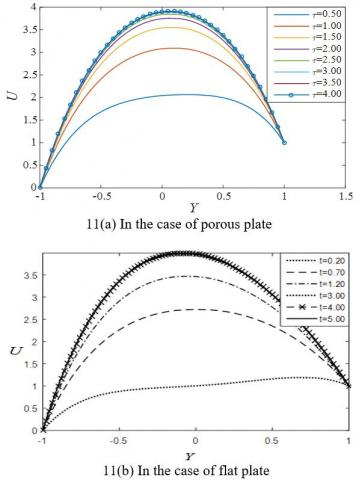Figure 11. Comparison with published results

Figure 11 shows that, both the researches show qualitatively quite same results. Quantitatively, the present results are little different due to the consideration of porous plate.

5. Conclutions

The MHD generalized Couette flow and heat transfer on Bingham fluid through porous parallel plates with Ion-slip and Hall currents has been investigated numerically by explicit finite difference scheme. The mesh sensitivity and time sensitivity tests are performed for obtaining appropriate mesh size and the steady-state solution respectively. The results were discussed for some important parameters such as Hall parameter $\left(\beta_{e}\right)$, Ion-slip parameter $\left(\beta_{i}\right)$ and Reynolds number $\left(R_{e}\right)$ and their effects on the flow behaviour. The most important outcomes of this investigation can be concluded as follows:

1. The steady-state solutions are obtained for the dimensionless time, $\tau =$4.00.
2. The obtained appropriate mesh size is $\left( m,n \right)\text{=}\left( \text{40,40} \right)$.
3. The primary velocity and temperature profiles increases with the increment of ${{\beta }_{e}}$, ${{\beta }_{i}}$ and ${{R}_{e}}$.
4. The secondary velocity increases with the increment of ${{R}_{e}}$ while it decreases with the rise of  ${{\beta }_{e}}$ and ${{\beta }_{i}}$ both.
5. The local Nusselt number decreases with the increment of ${{\beta }_{e}}$, ${{\beta }_{i}}$ and ${{R}_{e}}$.
6. The local shear stress increases with the increase of ${{\beta }_{e}}$, ${{\beta }_{i}}$ and ${{R}_{e}}$.
Nomenclature
 $\tilde{u},\tilde{w}$ primary and secondary velocity components ${{T}_{1}},\text{ }{{T}_{2}}$ temperatures at lower and upper plates ${{T}_{m}}$ non-dimensional mean fluid temperature $\rho$ density of the ﬂuid ${{B}_{0}}$ Uniform magnetic field $\tilde{\mu }$ Viscosity $\sigma$ electric conductivity of the fluid $k'$ magnetic permeability $\kappa$ thermal conductivity ${{c}_{p}}$ speciﬁc heat at the constant pressure $U,W$ dimensionless Primary and secondary velocity components $\theta$ dimensionless temperature $\tau$ dimensionless time ${{\tau }_{w}}$ dimensionless local shear stress at moving plate ${{N}_{u}}$ dimensionless local Nusselt number at moving plate ${{\tau }_{D}}$ Bingham number or dimensionless yield stress ${{\beta }_{e}}$ Hall parameter ${{\beta }_{i}}$ Ion-slip parameter ${{R}_{e}}$ Reynolds number ${{P}_{r}}$ Prandtl number ${{E}_{C}}$ Eckert number $Ha$ Hartmann number ${{k}_{0}}$ permeability of porous medium
References

 Bingham, E.C. (1916). An investigation of the laws of plastic flow. US Bureau of Standards Bulletin, 13: 309-353. https://doi.org/10.6028/bulletin.304

 Buckingham, E. (1921). On plastic flow through capillary tubes. ASTM Proceedings, 21: 1154-1156.

 Bingham, E.C. (1922). Fluidity and Plasticity. New York: McGraw-Hill, p. 219.

 Sakiadis, B.C. (1961). Boundary layer behavior on continuous solid surfaces: Boundary layer equations for two dimensional and axisymmetric flow. AICHE Journal, 7: 26-28. https://doi.org/10.1002/aic.690070108

 Soundalgekar, V.M., Murty, T.V.R. (1980). Heat transfer in flow past a continuous moving plate with variable temperature. Wärme - und Stoffübertragung, 14(2): 91-93. https://doi.org/10.1007/BF01806474

 Darby, R., Melson, J. (1981). How to predict the friction factor for flow of Bingham plastics. Chemical Engineering, 28: 59-61.

 Attia, H.A., Sayed-Ahmedm, M.E. (2004). Hall effect on unsteady MHD Couette flow and heat of a Bingham fluid with suction and injection. Applied Mathematical Modeling, 28: 1027-1045. https://doi.org/10.1016/j.apm.2004.03.008

 Liu, K.F., Mei, C.C. (2006). Slow spreading of a sheet of Bingham fluid on an inclined plane. Journal of Fluid Mechanics, 207: 505-529. https://doi.org/10.1017/S0022112089002685

 Naik, S.H., Rao, K.R., Murthy, M.V.R. (2014). The effect of hall current on unsteady MHD free convective Couette flow of a Bingham fluid with thermal radiation. International Journal of Engineering and Advanced Technology (IJEAT), 3(6): 1-16.

 Parvin, A., Dola, T.A., Alam, M.M. (2014). Unsteady MHD Bingham fluid flow with hall current and suction. International Journal of Modern Embedded System (IJMES), 2(5): 20-25.

 Rees, D.A.S., Bassom, A.P. (2015). Unsteady thermal boundary layer flows of a Bingham fluid in a porous medium. International Journal of Heat and Mass Transfer, 82: 460-467. https://doi.org/10.1016/j.ijheatmasstransfer.2014.10.047

 Hossain, M.D., Samad, M.A., Alam, M.M. (2016). MHD free convection and mass transfer flow through a vertical oscillatory porous plate in a rotating porous medium with hall, ion-slip currents and heat source. AMSE Journals -Series: Modelling B, 85(1): 28-42.

 Islam, M.M., Mollah, M.T., Hasan, M.S., Alam, M.M. (2017). Numerical solution of unsteady viscous compressible fluid flow along a porous plate with induced magnetic field. AMSE Journals-AMSE IIETA publication-2017-Series: Modelling B, 86(4): 850-863. https://doi.org/10.18280/mmc_b.860403

 Mollah, M.T., Islam, M.M., Alam, M.M. (2018). Hall and Ion-slip effects on unsteady MHD Bingham fluid flow with suction. Modelling, Measurement and Control B, 87(4): 221-229. https://doi.org/10.18280/mmc_b.870402

 Mollah, M.T., Islam, M.M., Alam, M.M. (2018). Couette flow of Bingham fluid with Ion-slip and hall current. Lambert Academic Publishing.

 Mollah, M.T. (2019). EMHD laminar flow of Bingham fluid between two parallel Riga plates. International Journal of Heat and Technology, 37(2): 641-648. https://doi.org/10.18280/ijht.370236

 Mollah, M.T., Islam, M.M., Ferdows, M., Alam, M.M. (2019). Bingham fluid flow through oscillatory porous plate with ion-slip and hall current. AIP Conference Proceedings, 2121(1): 050011. https://doi.org/10.1063/1.5115898

 Islam, M.M., Mollah, M.T., Khatun, S., Ferdows, M., Alam, M.M. (2019). Unsteady viscous incompressible Bingham fluid flow through a parallel plate. Inventions, 4(3): 51. https://doi.org/10.3390/inventions4030051

 Akter, M.S., Islam, M.R., Mollah, M.T., Alam, M.M. (2019). Hall effects on Casson fluid flow along a vertical plate. In AIP Conference Proceedings, 2121(1): 040004. https://doi.org/10.1063/1.5115875

 Islam, M.M., Khatun, S., Mollah, M.T., Alam, M.M. (2019). Fluid flow along the Riga plate with the influence of magnetic force in a rotating system. AIP Conference Proceedings, 2121(1): 050002. https://doi.org/10.1063/1.5115889